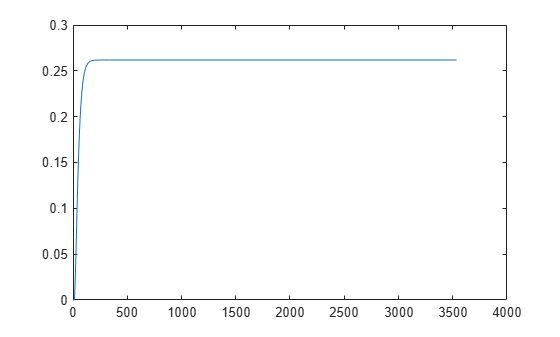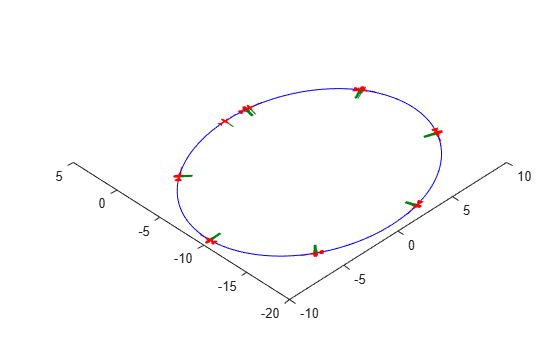state

UAV state vector

Description

example

stateVec = state(uavGuidanceModel) returns a state vector for the specified UAV guidance model. The vector is always filled with zeros. Use this function to ensure you have the proper size for your state vector. Use the state vector as an input to the derivative function or when simulating the UAV using ode45.

Examples

collapse all

This example shows how to use the multirotor guidance model to simulate the change in state of a UAV due to a command input.

Create the multirotor guidance model.

model = multirotor;

Create a state structure. Specify the location in world coordinates.

s = state(model);
s(1:3) = [3;2;1];

Specify a control command, u, that specified the roll and thrust of the multirotor.

u = control(model);
u.Roll = pi/12;
u.Thrust = 1;

Create a default environment without wind.

e = environment(model);

Compute the time derivative of the state given the current state, control command, and environment.

sdot = derivative(model,s,u,e);

Simulate the UAV state using ode45 integration. The y field outputs the multirotor UAV states as a 13-by-n matrix.

simOut = ode45(@(~,x)derivative(model,x,u,e), [0 3], s);
size(simOut.y)
ans = 1×2

13        3536

Plot the change in roll angle based on the simulation output. The roll angle (the X Euler angle) is the 9th row of the simOut.y output.

plot(simOut.y(9,:))Plot the change in the Y and Z positions. With the specified thrust and roll angle, the multirotor should fly over and lose some altitude. A positive value for Z is expected as positive Z is down.

figure
plot(simOut.y(2,:));
hold on
plot(simOut.y(3,:));
legend('Y-position','Z-position')
hold offYou can also plot the multirotor trajectory using plotTransforms. Create the translation and rotation vectors from the simulated state. Downsample (every 300th element) and transpose the simOut elements, and convert the Euler angles to quaternions. Specify the mesh as the multirotor.stl file and the positive Z-direction as "down". The displayed view shows the UAV translating in the Y-direction and losing altitude.

translations = simOut.y(1:3,1:300:end)'; % xyz position
rotations = eul2quat(simOut.y(7:9,1:300:end)'); % ZYX Euler
plotTransforms(translations,rotations,...
'MeshFilePath','multirotor.stl','InertialZDirection',"down")
view([90.00 -0.60])This example shows how to use the fixedwing guidance model to simulate the change in state of a UAV due to a command input.

Create the fixed-wing guidance model.

model = fixedwing;

Set the air speed of the vehicle by modifying the structure from the state function.

s = state(model);
s(4) = 5; % 5 m/s

Specify a control command, u, that maintains the air speed and gives a roll angle of pi/12.

u = control(model);
u.RollAngle = pi/12;
u.AirSpeed = 5;

Create a default environment without wind.

e = environment(model);

Compute the time derivative of the state given the current state, control command, and environment.

sdot = derivative(model,s,u,e);

Simulate the UAV state using ode45 integration. The y field outputs the fixed-wing UAV states based on this simulation.

simOut = ode45(@(~,x)derivative(model,x,u,e), [0 50], s);
size(simOut.y)
ans = 1×2

8   904

Plot the change in roll angle based on the simulation output. The roll angle is the 7th row of the simOut.y output.

plot(simOut.y(7,:))You can also plot the fixed-wing trajectory using plotTransforms. Create the translation and rotation vectors from the simulated state. Downsample (every 30th element) and transpose the simOut elements, and convert the Euler angles to quaternions. Specify the mesh as the fixedwing.stl file and the positive Z-direction as "down". The displayed view shows the UAV making a constant turn based on the constant roll angle.

downsample = 1:30:size(simOut.y,2);
translations = simOut.y(1:3,downsample)'; % xyz-position
rotations = eul2quat([simOut.y(5,downsample)',simOut.y(6,downsample)',simOut.y(7,downsample)']); % ZYX Euler
plotTransforms(translations,rotations,...
'MeshFilePath','fixedwing.stl','InertialZDirection',"down")
hold on
plot3(simOut.y(1,:),-simOut.y(2,:),simOut.y(3,:),'--b') % full path
xlim([-10.0 10.0])
ylim([-20.0 5.0])
zlim([-0.5 4.00])
view([-45 90])
hold offInput Arguments

collapse all

UAV guidance model, specified as a fixedwing or multirotor object.

Output Arguments

collapse all

State vector, returned as a seven-element or thirteen-element vector. The vector is always filled with zeros. Use this function to ensure you have the proper size for your state vector.

For fixed-wing UAVs, the state is an eight-element vector:

• North - Position in north direction in meters.

• East - Position in east direction in meters.

• Height - Height above ground in meters.

• AirSpeed - Speed relative to wind in meters per second.

• HeadingAngle - Angle between ground velocity and north direction in radians.

• FlightPathAngle - Angle between ground velocity and north-east plane in radians.

• RollAngle - Angle of rotation along body x-axis in radians.

• RollAngleRate - Angular velocity of rotation along body x-axis in radians per second.

For multirotor UAVs, the state is a thirteen-element vector in this order:

• World Position - [x y z] in meters.

• World Velocity - [vx vy vz] in meters per second.

• Euler Angles (ZYX) - [psi theta phi] in radians.

• Body Angular Rates - [p q r] in radians per second.

• Thrust - F in Newtons.

Extended Capabilities

Blocks

Introduced in R2018b

UAV Toolbox DocumentationGet trial now